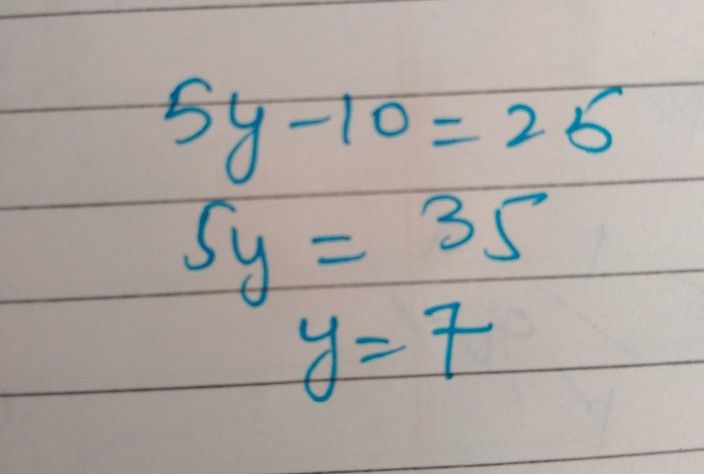Symbol
Problem$y$ $12$ $5y-10=35$
Algebra
SolutionQanda teacher - GaganStudent
find the simple interest for the priniciple rupees 500at the rate is of 5%2yearsQanda teacher - GaganStudent